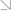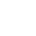全国热线：400-963-0708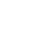网站地图 Sign in Sign up

# 备考FRM考试需要做FRM真题练习吗？

2021年新版FRM备考资料下载
• 考纲对比
• 学习计划
• 思维导图
• 复习资料
• 历年真题
• 词典及公式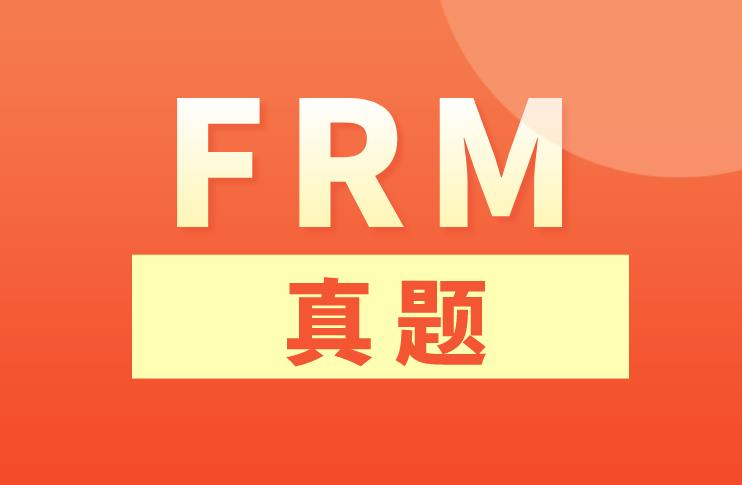ACRO of a hedge fund is asking the risk team to develop a term-structure model that is appropriate for fitting interest rates for use in the fund’s options pricing practice. The risk team is evaluating among several interest rate models with time-dependent drift and time-dependent volatility functions. Which of the following is a correct description of the specified model?

A) In the Ho-Lee model, the drift of the interest rate process is presumed to be constant.

B) In the Ho-Lee model, when the short-term rate is above its long-run equilibrium value, the drift is presumed to be negative.C) In the Cox-Ingersoll-Ross model, the basis-point volatility of the short-term rate is presumed to be proportional to the square root of the rate, and short-term rates cannot be negative.

D) In the Cox-Ingersoll-Ross model, the volatility of the short-term rate is presumed to decline exponentially to a constant level.

Analyst Barry runs a short-term interest rate simulation using Model 1, which assume no drift. The time step in his model is one month. His Model 1 also makes two assumptions. First, the initial or current short-term rate is equal to 4.00%. Second, the annual basis-point volatility is 200 basis points. In the first step of his first trial, the random uniform variable is 0.8925 such that, via inverse transformation, the associated random standard normal value is 1.240. To what level does the rate evolve in the first month?

A) 3.854%

B) 4.716%

C) 5.393%

D) 6.480%FRM学习交流群(扫码免费加群)

•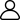1、新手入门
•2、学习资料
•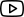3、免费课程
•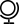4、考试动态
•5、备考干货
•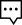6、答疑冲刺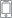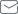2021年7月10日 FRM考试 还有

100
10小时 10分钟 10

## 推荐课程